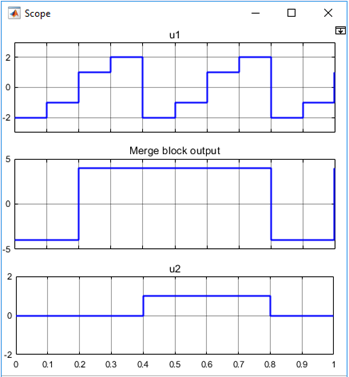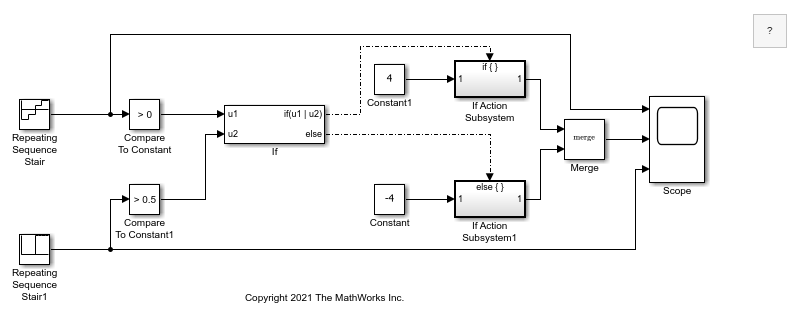## Fixed-Point Data Type Support for If Block

This topic explains how to use a Compare To Constant block for If block fixed-point data type support.

### Floating-Point Model without Fixed-Point Data

Open this floating-point model without fixed-point data types.

```open_system("ex_if_block_floatingpoint.slx"); ```### Block and Model Configurations

In this model, the If Action Subsystem blocks use their default configurations. The simulation parameters are set to their default values except for the parameters listed in this table.

Configuration Parameter Pane

Parameter

Setting

Solver

Start time

`0.0`

Stop time

`1.0`

Type

`Fixed-step`

Solver

```discrete (no continuous states)```

Fixed-step size

`0.1`

The block parameters are set to their default values except for the parameters listed in this table.

Block

Parameter

Setting

Repeating Sequence Stair

Vector of output values

`[-2 -1 1 2].'`

Repeating Sequence Stair1

Vector of output values

`[0 0 0 0 1 1 1 1].'`

If

Number of inputs

`2`

If expression

```(u1 > 0) | (u2 > 0.5)```

Show else condition

Selected

Constant

Constant value

`-4`

Constant1

Constant value

`4`

Scope

Number of axes

`3`

Time range

`1`

For this model, when input `u1` is greater than `0` or input `u2` is greater than `0.5`, the output is `4`. Otherwise, the output is `-4`. The Scope block displays the output from the Merge block with inputs `u1` and `u2`.### Model with If Action Subsystems and Fixed-Point Data Types

You can implement this block diagram as a model with fixed-point data types. Open this model with fixed-point data types.

```open_system("ex_if_block_fixedpoint.slx"); ```### Model Analysis

The Repeating Sequence Stair blocks output fixed-point data types.

The Compare To Constant blocks implement two parts of the If expression that is used in the If block in the floating-point version of the model, `(u1 > 0)` and `(u2 > 0.5)`. The `OR` operation, `(u1|u2)`, can still be implemented inside the If block. For a fixed-point model, the expression must be partially implemented outside of the If block as it is in this model.

The block and simulation parameters for the fixed-point model are the same as for the floating-point model with these exceptions and additions.

Block

Parameter

Setting

Compare To Constant

Operator

`>`

Constant value

`0`

Output data type mode

`Boolean`

Enable zero-crossing detection

off

Compare To Constant1

Operator

`>`

Constant value

`0.5`

Output data type mode

`Boolean`

Enable zero-crossing detection

off

If

Number of inputs

`2`

If expression

`u1|u2`